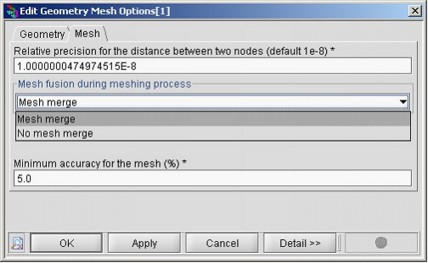# Geometry and mesh options

## Activation

There are two ways to get access to the window for geometry and mesh options:• in the menu bar select Tools and click Geometry and Mesh options and then Edit
• in the data tree, in the folder Tools, edit the Geometry and Mesh options entity

## Geometry options

Only one geometry option can be changed by the user:

• the relative precision for the distance between two points (also called relative epsilon)

It makes it possible to compute the minimum distance between two points that separates two distinguished points. Below this distance Flux PEEC forbids the creation of the second point.

The calculation of this distance is done by the formula: minimum distance = relative precision * diagonal

where

diagonal: represents the diagonal of the plane-parallel box surrounding the system being modeled.

The default value of the relative precision is 10-8.

## Mesh options

Three mesh options can be changed by the Flux PEEC user:

• the relative precision (also called relative epsilon) for the distance between two nodes

It makes it possible the calculation of the minimum distance between two different mesh nodes. Below this value both nodes would join which is forbidden by Flux PEEC.

The minimum distance is given by the formula: minimum distance = relative precision * diagonal

where

diagonal: represents the diagonal of the plane-parallel box surrounding the system being modeled.

The default value of the relative precision is 10-8.

The relative precision of the mesh must be smaller or equal the relative precision of the geometry, i.e. two geometric points cannot be closer than two mesh nodes.

• the mesh fusion during the meshing process

By default, the automatic mesh algorithm according to the frequency searches some mesh elements to join in the unidirectional conductors: the mesh elements that can be merged are two adjacent rectangular mesh elements that do not belong to the skin depth and whose length or width is equal.

The user can disable this fusion option, leading to an increase of the amount of mesh elements without particular improvement of the result quality.

• the accuracy of the mesh expressed in percentage
• The algorithms for uniform mesh and for automatic mesh according to frequency include a refinement step on the edges for unidirectional conductors of complex shape in order to fit better to the geometry. This refinement step stops when the difference between total surface of the mesh elements contained in the profile and the total surface of these mesh elements added to those that would straddle the contour is lower than the accuracy defined.

The default value for the accuracy is set to 5% in Flux PEEC.

The value chosen applies to all the conductors. It is not possible to set a different value for each conductor.